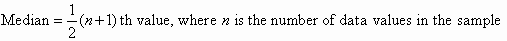# what is the formula of mean , median , mode ?

Mean

The mean (or average) of a set of data values is the sum of all of the data values divided by the number of data values.

That is:### Median

The median of a set of data values is the middle value of the data set when it has been arranged in ascending order.

That is, from the smallest value to the highest value.

In General;

When n = no. of values is odd,and

When n =  number of values in the data set is even, then the median is the average of the two middle values.### Mode

The mode of a set of data values is the value(s) that occurs most often.

The formula used to find the mode when the data is given in groups is as follows.

 Mode =Where, l = lower limit of the modal class

h = size of class interval (all class sizes should be equal)

f1 = frequency of the modal class

f0 = frequency of the class preceding the modal class

f2 = frequency of the class succeeding the modal class

• 48

mean=  sum.of all observations/No.of observations

median=  arrange the observations in either ascending or descending order.Then find the middle value of the distribution.

mode=  value of observation which occurs most frequently

................THUMBS UP pls

• 22

mean : xbar = sum of all obsv. / no. of . obsv .

median : odd  { x+1/2 }

even (x+2)+(x/2+1)/2

mode : the obsv. which has the highest frequency represents the mode .

• -3

u knew da answer or wat??

• -18

i do not know

• -18

from mcgs

• -16

MEAN IS THE AVERAGE OF ALL OBSERVATIONS

MEAN = SUM OF OBSERVATIONS / TOTAL NUMBER OF OBSERVATIONS( n )

MEDIAN IS THE MIDDLE MOST OBSERVATION WHEN TH DATA IS ARRANGED IN ASCENDING OR DESCENDING ORDER

MEDIAN = [ ( n + 1 ) / 2 ] th OBSERVATION IF IT IS ODD AND [ ( n / 2 ) th OBSERVATION + { ( n / 2 ) + 1 } th OBSERVATION ] / 2

MODE IS THE OBSERVATION WHICH OCCURS FOR THE MOST TIMES IN AN DATA

THERE IS ALSO A RELATION BETWEEN THEM ,

MODE = 3 MEDIAN - 2 MEAN

• 2
What are you looking for?